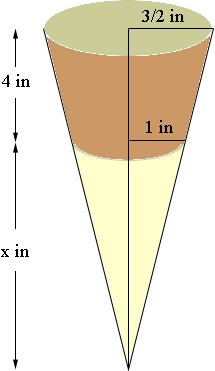SEARCH HOMEMath Central Quandaries & QueriesQuestion from leanna, a student: find the volume of a styrofoam cup if the diameter of the top is three inches, the diameter of the base is 2 inches, and the height is 4 inches.Hi Leanna,

The shape you describe is called a truncated cone. If you first need to extend the cup downwards to the apex of the cone. I said that the length of this extension is x inches.The cup has a radius of 3/2 inches at the top and 1 inch at the bottom. You can then find the volume of the cup by finding the volume of a cone of radius 3/2 inches and height (4 + x) inches and subtracting the volume of a cone of radius 1 inch and height x inches. All that remains is to find the value of x.

To see how to find the value of x go to the Quandaries and Queries section of Math Central and search in the Quick Search area using the term truncated cone. In the list of item that is returned you will see how to do this.

PennyMath Central is supported by the University of Regina and The Pacific Institute for the Mathematical Sciences.